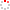#CodeStepByStep## reverse_chunks_in_file

Language/Type: Python file input

Write a function named `reverse_chunks_in_file` that accepts a `string` parameter representing an input file name, where the file is filled with s/k word/integer pairs on each line, and reverses the chunks of each word, printing the words to the console. The "reversing of chunks" is as defined in the separate string problem `reverse_chunks` found elsewhere in this site. (See that problem for details.) For example, if the file `myinput.txt` contains the following text:

```MehranSahami 3 ChrisP 2
MartyStepp 4 KeithSchwarz 5 CynthiaBLee 3

NickT 1
```

Then the call of `reverse_chunks_in_file("myinput.txt")` should print the following console output:

```heMnarhaSima hCirPs
traMetSypp htieKawhcSrz nyCihtLBaee

NickT
```

Notice that each word has its chunks reversed using the k value that immediately follows that word, such as the `3` after `"MehranSahami"` or the `5` after `"KeithSchwarz"`. You must retain the line breaks that were present in the file originally.

Assumptions: You may assume that the given file exists, is readable, and follows the given format with an alternating pattern of words and integers. You may assume that the integers that follow each word are positive numbers. You may assume that each word on each line of the file is separated by a single space.

Constraints: In solving this problem, you should not create any data structures such as lists. But you may create as many strings as you like, and you may use as many simple variables (such as `int`s) as you like.

Note: You may want to go solve the string problem `reverse_chunks` first and then paste its solution here so that you can call it as a helper to solve this problem. You can submit both functions in your solution, one after the other.

Type your Python solution code here:

This is a function exercise. Write a Python function as described. Do not write a complete program; just the function(s) above.